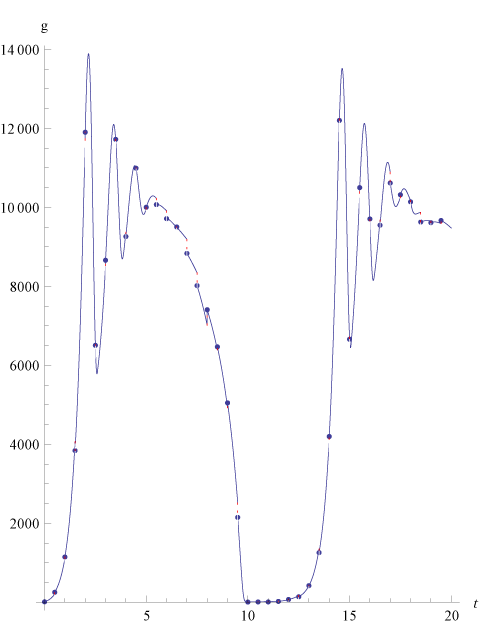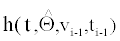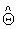Figure 5: Representation of partially observed Calcium oscillation system’s dynamics, g. The blue points are the data points. Blue curves are the ODE dynamics for each time interval [ti-1,ti] namelywith the estimated parameter. Red dotted lines show the residuals. 100 observation from from partially observed Calcium oscillation model (g) with T=50 and v0=(10,10,10) for the estimation ofusing (2) with K={0}. Dynamics shown until T=20.